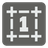The Hamming Distance
Elementary
English FR HU RU UK

The Hamming distance between two binary integers is the number of bit positions that differs (read more about the Hamming distance on Wikipedia). For example:

```    117 = 0 1 1 1 0 1 0 1
17 = 0 0 0 1 0 0 0 1
H = 0+1+1+0+0+1+0+0 = 3

```

You are given...

You should be an authorized user in order to see the full description and start solving this mission.